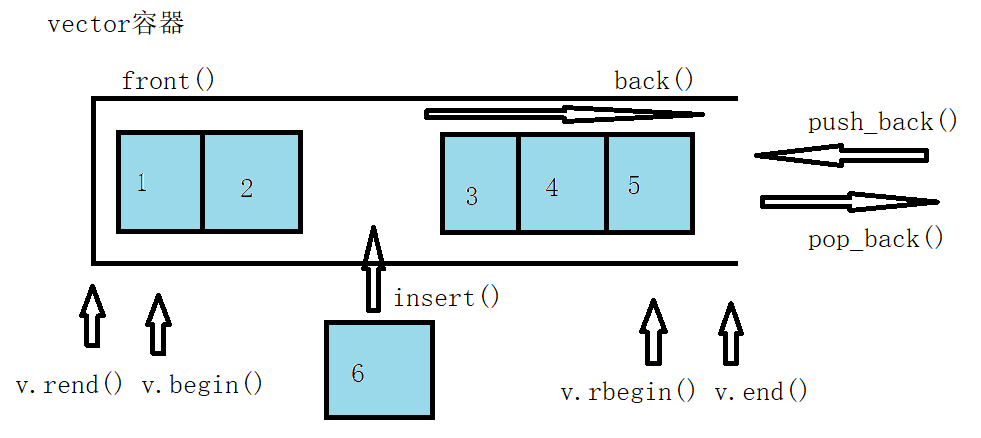# vector

vector数据结构和数组非常相似，也称为单端数组
vector与普通数组区别： 不同之处在于数组是静态空间，而vector可以动态扩展
vector容器的迭代器是支持随机访问的迭代器## vector构造函数

vector<T> v;                      //采用模板实现类实现，默认构造函数
vector(v.begin(), v.end());       //将v[begin(), end())区间中的元素拷贝给本身。
vector(n, elem);                  //构造函数将n个elem拷贝给本身。
vector(const vector &vec);        //拷贝构造函数


## vector赋值操作

vector& operator=(const vector &vec); //重载等号操作符
assign(beg, end);       			  //将[beg, end)区间中的数据拷贝赋值给本身。
assign(n, elem);       			      //将n个elem拷贝赋值给本身。


## vector容量和大小

empty(); 				//判断容器是否为空
capacity(); 			//容器的容量
size(); 				//返回容器中元素的个数
resize(int num); 		//重新指定容器的长度为num，若容器变长，则以默认值填充新位置。
//如果容器变短，则末尾超出容器长度的元素被删除。
resize(int num, elem);  //重新指定容器的长度为num，若容器变长，则以elem值填充新位置。
//如果容器变短，则末尾超出容器长度的元素被删除


## vector插入和删除

push_back(ele);                                     //尾部插入元素ele
pop_back();                                         //删除后一个元素
insert(const_iterator pos, ele);        			//迭代器指向位置pos插入元素ele
insert(const_iterator pos, int count,ele); 			//迭代器指向位置pos插入count个元素ele
erase(const_iterator pos);                     		//删除迭代器指向的元素
erase(const_iterator start, const_iterator end);	//删除迭代器从start到end之间的元素
clear();                                            //删除容器中所有元素


## vector数据存取

at(int idx);     //返回索引idx所指的数据
operator[];      //返回索引idx所指的数据
front();         //返回容器中第一个数据元素
back();          //返回容器中后一个数据元素


## vector互换容器

swap(vec);  // 将vec与本身的元素互换


#include <iostream>
#include <vector>

using namespace std;

int main()
{
vector<int> v;
for (int i = 0; i < 100000; i++)
{
v.push_back(i);
}

cout << "v的容量为：" << v.capacity() << endl;
cout << "v的大小为：" << v.size() << endl;

v.resize(3);

cout << "v的容量为：" << v.capacity() << endl;
cout << "v的大小为：" << v.size() << endl;

//收缩内存
vector<int>(v).swap(v); //匿名对象

cout << "v的容量为：" << v.capacity() << endl;
cout << "v的大小为：" << v.size() << endl;
return 0；
}


## vector预留空间

reserve(int len); //容器预留len个元素长度，预留位置不初始化，元素不可访问


03-28627
01-155万+03-01680
10-19341
03-01452
08-10649
07-141003
03-051296
12-1410万+
11-237034